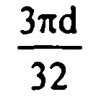Search

# Fluid Mechanics (1-20)

Updated: Apr 5, 2020

1. Pascal-second is the unit of - dynamic viscosity

2. An ideal fluid is- frictionless and incompressible

3. The unit of kinematic viscosity is-cm2/sec

4. If the dynamic viscosity of a fluid is 0.5 poise and specific gravity is 0.5, then

the kinematic viscosity of that fluid in stokes is-1.0

5. The viscosity of a gas - increases with increase in temperature

6. Newton's law of viscosity relates - shear stress and rate of angular deformation

7. The rise of liquid of specific weight y in a capillary tube of radius r is given by8. The intensity of pressure developed by surface tension of 0.075 N/m in a

droplet of water of 0.075 mm diameter - 0.4 N/cm2

9. Surface tension of water- increases with decrease in temperature

10. One kilo-Pascal is equivalent to- 0.001 N/mm2

11. If a liquid has greater cohesion than adhesion with the solid, then the liquid in

the capillary tube will- depress with convex surface upward

12. Surface tension is due to cohesiononly, Capillarity is due to both cohesion and adhesion

13. Pressure of 200 kPa is equivalent to a head of x metres of carbon tetra-chloride

of relative density 1.59 where x is equal to- 12.82

14. For a vertical semi-circular plate, sub merged in a homogenous liquid with its

diameter 4d' at the free surface, the depth of centre of pressure from the free surface is-15. The pressure intensity is same in all directions at a point-when there is no relative motion of one fluid layer relative to other

16. An open tank contains 1 m deep water with 50 cm depth of oil of specific gravity 0.8 above it. The intensity of pressure at the bottom of tank will be-14 kN/m2

17. The position of centre of pressure on a plane surface immersed vertically in a

static mass of fluid is- always below the centroid of the area

18. A vertical triangular area with vertex downward and altitude 'h' has its base

lying on the free surface of a liquid. The centre of pressure below the free surface

is at a distance of- h/2

19. The total pressure on a plane surface inclined at an angle 6 with the horizontal

is equal to-pA

20. A vertical rectangular plane surface is submerged in water such that its top and bottom surfaces are 1.5 m and 6.0 m res pectively below the free surface. The

position of centre of pressure below the free surface will be at a distance of -4.2m